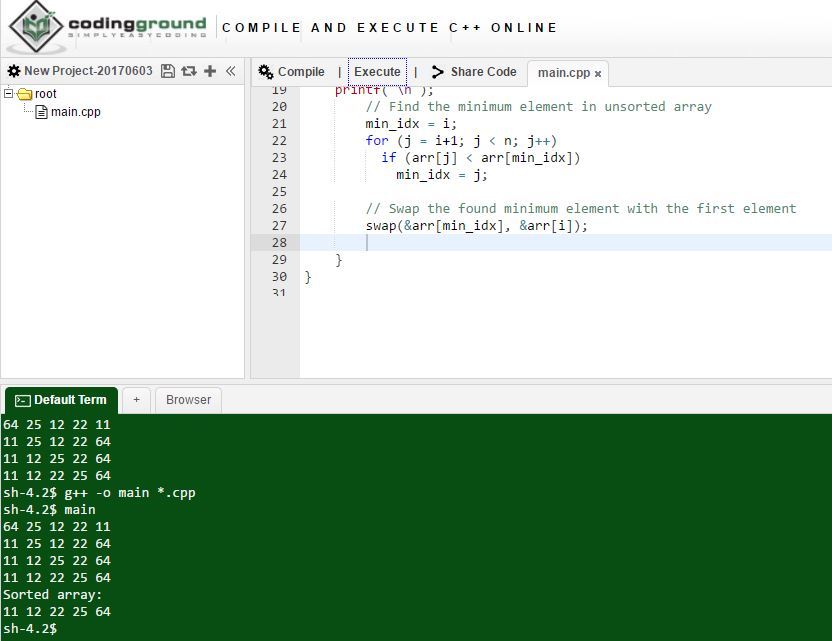# Answered! 1. Sort the following list using the selection sort algorithm as discussed in this chapter. Show the list…

1. Sort the following list using the selection sort algorithm as discussed in this chapter. Show the list

after each iteration of the outer for loop.

Don't use plagiarized sources. Get Your Custom Essay on
Answered! 1. Sort the following list using the selection sort algorithm as discussed in this chapter. Show the list…
GET AN ESSAY WRITTEN FOR YOU FROM AS LOW AS \$13/PAGE

36, 55, 17, 35, 63, 85, 12, 48, 3, 66

2. Given the declaration:

char string15;

Mark the following statements as valid or invalid. If a statement is invalid, explain why.

a. strcpy(string15, “Hello there”);

b. strlen(string15);

c. string15 = “Jacksonville”;

d. cin >> string15;

e. cout << string15;

f. if

(string15 >= “Nice day”)

cout << string15;

g. string15 = ‘t’;

3. Given the declaration:

char name = “Shelly”;

Mark the following statements as ‘‘Yes’’ if they output Shelly. Otherwise, mark the

statement as ‘‘No’’ and explain why it does not output Shelly.

a. cout << name;

b. for(int j = 0; j < 6; j++)

cout << name[j];

c. int j = 0;

while(name[j] != ‘’)

cout << name[j++];

d. int j = 0;

while(j < 8)

cout << name[j++];

Hi,

Ans 1. Selection sort algorithm in C++ with each step of iteration-

C++ code-

#include <stdio.h>

void swap(int *xp, int *yp)
{
int temp = *xp;
*xp = *yp;
*yp = temp;
}

void selectionSortAlgo(int arr[], int n)
{
int i, j, min_idx;
for (i = 0; i < n-1; i++)
{int k;
for (k=0; k < n; k++)
printf(“%d “, arr[k]);
printf(“n”);
min_idx = i;
for (j = i+1; j < n; j++)
if (arr[j] < arr[min_idx])
min_idx = j;

swap(&arr[min_idx], &arr[i]);

}
}

void Display(int arr[], int size)
{
int i;
for (i=0; i < size; i++)
printf(“%d “, arr[i]);
printf(“n”);
}

// Driver program to test above functions
int main()
{
int arr[] = {64, 25, 12, 22, 11};
int n = sizeof(arr)/sizeof(arr);
selectionSortAlgo(arr, n);
printf(“Sorted array: n”);
Display(arr, n);
return 0;
}

Screenshot-Ans 2- Please find below the answers for all the sub questions-

a) char string15;
strcpy(string15, “Hello there”); //valid as the char array lenth is 16 and the characters that are copied have length 11
b)

string15 = ‘Jacksonville’;//incompatible types in assignment of ‘const char ’ to ‘char ’
c)

cin>>string15; //This statement takes the char array as an input and hence it is a valid statement
d)

cout<<string15; // This statement prints out the char array as output as it is a standard output
e)

if
(string15 >= “Nice day”) // This is a valid statement as it compares length of the string ‘string15’ with “Nice Day”
cout << string15;
f)

string15 = ‘t’; // This statement is valid as it assigns the value ‘t’ to the ndex location 6 of the char array string15

a. cout << name; //Yes
b. for(int j = 0; j < 6; j++) //Yes
cout << name[j];
c. int j = 0;
while(name[j] != ‘’)
cout << name[j++]; //Yes
d. int j = 0;
while(j < 8)
cout << name[j++]; //Yes

Thanks and Regards,

Vinay Prakash Singh